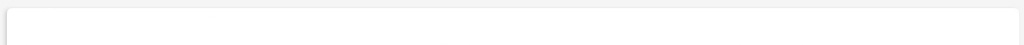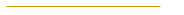ClassDivulgationHomeNotesPhotographsPress articles about
research work and conferencesPrizesPrizes for thesis under supervisionPublicationsSeminars and ConferencesSoftwareThesis under my supervision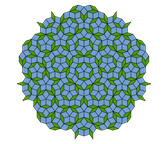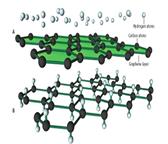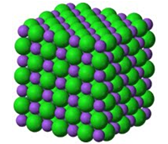Contact Information

Email
naumis@fisica.unam.mx

Fax
(525) 5622-50-08

Room 37,
Main Building,
Institute of Physics,
C.U., México D.F.

Apdo. Postal 20-364, 01000, México D.F., México.

Software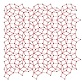1. QPtilingApproximants
This program calculate the vertex positions of 2D quasiperiodic tilings APPROXIMANTS using the generalized dual method.

It is just a simple program from were you can start other applications like the 3D version (it is just very easy to do,from the present code). First, you give the star vectors e_{i}, i.e. a set of unitary vectors that have the required symmetry. For example, in the Penrose it is required 5 fold symmetry, thus, the star is a set of 5 vectors that point to the vertex of a pentagon. Then, all intersections of a family of lines perpendicular to each vector is calculated. Following the formulas of G.G. Naumis, J.L. Aragón,"Analytic expressions for the vertex coordinates of quasiperiodic lattices",Z. Kristallogr. 218, 397 (2003) which should be cited when this program is used. This program has been made by Gerardo G. Naumis and José Luis Aragon, in 2002, at the Instituto de Fisica, National University of Mexico, and modified in 2015 to include approximants. To draw the tiling, just make a program that joins all points separated by a distance "1". See our mathematica version of the program in the same webpage: www.fisica.unam.mx/naumis APPROXIMANTS are computed by using rational approximants for the star vectors For a Penrose, this is done by using a basis star which contains Fibonacci number approximants to the golden mean. Here we present two options as in the program. One gives a rectangular cell (ortorhombic) unit cell, while the other can give you a skkiny or fat rhombus unit cell. See documentation inside the program on how to use this options. For other rotational symmetries, one needs to apply a similar procedure. If you have comments and questions, contact: G. Naumis naumis@fisica.unam.mx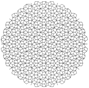2. Averagero
Program in Mathematica, draw quasiperiodic, specially Penrose tilings using a simple interface.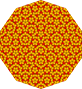3. Penrose
Same very simple program in FORTRAN 77 to generate Penrose and other quasiperiodic tilings. Contains documentation and FAQ about tilings.

THESE ARE THE SIMPLEST PROGRAMS TO GENERATE QUASIPERIODIC TILINGS. As default parameters, the programs generate a Penrose tiling, but other isomorphism classes are available by changing the parameters. The program gives some pictures of the generated tilings, and outputs a file with the VERTEX positions. Written as a Notebook in MATHEMATICA and FORTRAN, the programs also allow to obtain the average structure and the corresponding diffraction pattern. Made from our analytical recipe to get the vertex positions as described in: "Analytical expressions for the vertex coordinates of quasiperiodic lattices", by G. Naumis, J.L. Aragón, Z. Kristallogr. (2003) 218 397, which to our knowledge is the only analytical formula to get the positions in a quasicrystal without any search procedure; the formula is just a generalization of how the vertex positions are found in a crystal: by making integer linear combinations of basis vectors. The simple trick is to use a floor function to generate these linear combinations. Many important results are obtained using this recipe (see G.G. Naumis, "Phason hierarchy and electronic stability of quasicrystals", Phys, Rev. B71, 144204 (2005). It can be easily generalized in 3 dimensions. Important notice: each time that the parameters are changed in the Mathematica version, one needs to quit and run mathematica again. This bug soon will be fixed.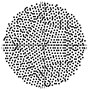4. Cass2: Notebook in MATHEMATICA for generating quasiperiodic lattices in 2dimensions using the most general analytical expressions that were developed in: "Analitical expressions for the vertex coordinates of quasiperiodic lattices", G.G. Naumis, J.L. Aragón, Z. Kristallogr. (2003) 218 397. Therein you will find the notation and the algorithm. This program contains Averagero as a particular case, but contains much more parameters and thus freedom. This program is able to generate very rare and interesting lattices!! Important notice: each time that the parameters of the grids are changed, one needs to quit and run mathematica again. This bug soon will be fixed.